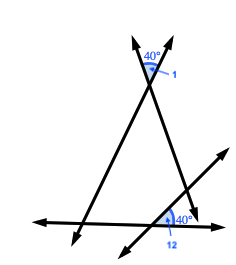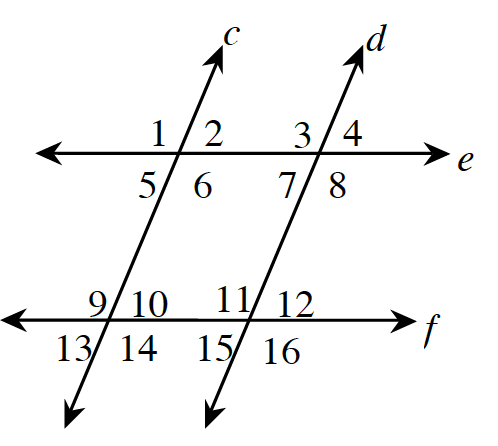### Home > CCAA > Chapter 9 Unit 10 > Lesson CC3: 9.1.3 > Problem9-33

9-33.

Based on the given information, determine which pairs of lines, if any, are parallel. If none are necessarily parallel, write “none.”

Alternate interior angles and corresponding angles have equal measure only if the lines cut by the transversal are parallel.
Conversely, if the angle measures are equal, the lines must be parallel.

1. $m∠2 = m∠7$

These are alternate interior angles.

2. $m∠3 = m∠11$

These are corresponding angles.

$e$ and $f$

3. $m∠1 = m∠12$

In the diagram below, the measures of $\angle1$ and $\angle12$ are equal. Do any two lines look parallel?4. $m∠13 = m∠12$

Vertical angles always have equal measure.

$c$ and $d$

5. $∠6$ and $∠7$ are supplementary.

$\angle6$ would be supplementary to $\angle7$ if $m\angle7$ was equal to $m\angle2$.